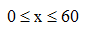# Interval Notation: Definition & Examples

Instructions:

Choose an answer and hit 'next'. You will receive your score and answers at the end.

question 1 of 3

### Identify the type of interval (0,2]:

Create Your Account To Take This Quiz

As a member, you'll also get unlimited access to over 75,000 lessons in math, English, science, history, and more. Plus, get practice tests, quizzes, and personalized coaching to help you succeed.

Try it risk-free for 30 days. Cancel anytime.

### 2. Write the following inequality in interval notation:Create your account to access this entire worksheet
A Premium account gives you access to all lesson, practice exams, quizzes & worksheets
Quizzes, practice exams & worksheets
Certificate of Completion
Create an account to get started

Interval notation is an important strategy that you can use to simplify problems and information. This quiz/worksheet combo will help you test your understanding of interval notation, and the practice questions will test you on identifying the types of intervals and writing problems in interval notation.

## Quiz & Worksheet Goals

In these assessments, you'll be tested on:

• Identifying types of intervals
• Rewriting inequalities in interval notation
• Defining intervals from graphed inequalities

## Skills Practiced

This quiz and worksheet will allow you to test the following skills:

• Reading comprehension - ensure that you draw pertinent information from the related lesson on interval notation
• Making connections - use interval notation to simplify inequalities
• Problem solving - use your acquired knowledge to solve practice problems involving inequalities and intervals

If you would like to study more, review the accompanying lesson called Interval Notation: Definition & Examples. This lesson covers the following objectives:

• Define interval notation
• Describe how to use interval notation
• Differentiate between open and closed intervals
Final ExamGeneral Studies Math: Help & Review
Status: Not Started
Chapter ExamData, Statistics & Probability Fundamentals
Status: Not Started

Support